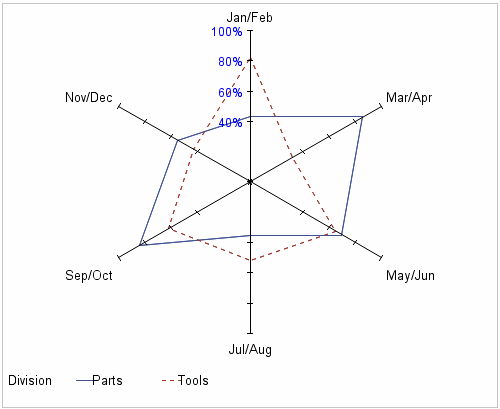# Example 6: Modifying Chart Axes

 Features: AXIS statement options: ORDER= VALUE= CHART statement options: HEIGHT= NOFRAME STARAXIS= STARINRADIUS= SUMVAR= FORMAT procedure Sample library member: GRRAXIS
You can use AXIS statements to modify the tick marks that appear on the chart spokes and the order in which the tick mark labels appear. This example uses AXIS statements, in addition to formats, to modify the tick marks, tick mark labels, and axis labels on the radar chart spokes.## Program

```libname library "library-specification";
proc format library=library;
value mnth
1="Jan/Feb" 2="Mar/Apr" 3="May/Jun"
4="Jul/Aug" 5="Sep/Oct" 6="Nov/Dec";
run;```
```data goals;
input month Division \$ value @@;
format month mnth.;
format value percentn7.0;
datalines;
1 Parts .43 1 Tools .82
2 Parts .86 2 Tools .32
3 Parts .70 3 Tools .65
4 Parts .35 4 Tools .52
5 Parts .84 5 Tools .62
6 Parts .55 6 Tools .43
;
run;```
`goptions reset=all border hsize=5.15in vsize=4.2in;`
```axis1 order=(0 to .4 by .4, .6 to 1 by .2) value=(height=3pct c=blue tick=1 "");
axis2 order=(0 to .4 by .4, .6 to 1 by .2) value=none;```
```proc gradar data=goals;
chart month / sumvar=value
staraxis=(axis1 axis2 axis2 axis2 axis2 axis2)
noframe height=3.25
overlayvar=division;
run;
quit;```

## Program Description

Create a user-defined format, MNTH, for the axis labels. Replace library-specification with the path to your library.
```libname library "library-specification";
proc format library=library;
value mnth
1="Jan/Feb" 2="Mar/Apr" 3="May/Jun"
4="Jul/Aug" 5="Sep/Oct" 6="Nov/Dec";
run;```
Create the input data set WORK.GOALS. The user-defined format is applied to the variable MONTH, and the SAS format PERCENTN7.0 is applied to the variable VALUE.
```data goals;
input month Division \$ value @@;
format month mnth.;
format value percentn7.0;
datalines;
1 Parts .43 1 Tools .82
2 Parts .86 2 Tools .32
3 Parts .70 3 Tools .65
4 Parts .35 4 Tools .52
5 Parts .84 5 Tools .62
6 Parts .55 6 Tools .43
;
run;```
Set the graphics environment.
`goptions reset=all border hsize=5.15in vsize=4.2in;`
Define tick marks for the chart spokes.The AXIS1 statement controls the tick marks for the first (12 o’clock) axis. The ORDER= option specifies the tick mark interval and the actual values that correspond to each the tick mark. The format PERCENTn7.0 that was applied to the variable VALUE in the DATA step controls how the values are displayed for the tick mark labels. The VALUE= option specifies the size and color of the tick mark labels. The AXIS2 statement controls all axes except the first. For those axes, the VALUE= option specifies that no tick mark labels are displayed.
```axis1 order=(0 to .4 by .4, .6 to 1 by .2) value=(height=3pct c=blue tick=1 "");
axis2 order=(0 to .4 by .4, .6 to 1 by .2) value=none;```
Create the radar chart. The STARAXIS= option tells the procedure to apply the AXIS1 statement to the first axis and the AXIS2 statement to all other axes in the chart.
```proc gradar data=goals;
chart month / sumvar=value
staraxis=(axis1 axis2 axis2 axis2 axis2 axis2)
noframe height=3.25